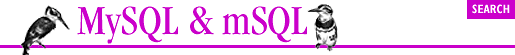home | O'Reilly's CD bookshelfs | FreeBSD | Linux | Cisco | Cisco Exam## 14.2. Simple Database Access

#### Example 14-1. Inserting a Row into mSQL Using a JDBC Statement Object

```import java.sql.*;
import java.util.*;

public class Insert {
// We are inserting into a table that has two columns: test_id (int)
// and test_val (char(55))
// args is the test_id and args the test_val
public static void main(String argv[]) {
Connection con = null;
ResourceBundle bundle = ResourceBundle.getBundle("SelectResource");

try {
String url = bundle.getString("URL");
Statement stmt;

Class.forName(bundle.getString("Driver"));
// here is where the connection is made
con = DriverManager.getConnection(url, "borg", "");
stmt = con.createStatement();
stmt.executeUpdate("INSERT INTO test (test_id, test_val) " +
"VALUES(" + args + ", '" + args + "')");
}
catch( SQLException e ) {
e.printStackTrace();
}
finally {
if( con != null ) {
try { con.close(); }
catch( Exception e ) { }
}
}
}
}```

#### Example 14-2. A Simple Query

```import java.sql.*;
import java.util.*;

public class Select {
public static void main(String argv[]) {
Connection con = null;
ResourceBundle bundle =
ResourceBundle.getBundle("SelectResource");

try {
String url = bundle.getString("URL");
Statement stmt;
ResultSet rs;

Class.forName(bundle.getString("Driver"));
// here is where the connection is made
con = DriverManager.getConnection(url, "borg", "");
stmt = con.createStatement();
rs = stmt.executeQuery("SELECT * from test ORDER BY test_id");
System.out.println("Got results:");
while(rs.next()) {
int a= rs.getInt("test_id");
String str = rs.getString("test_val");

System.out.print(" key= " + a);
System.out.print(" str= " + str);
System.out.print("\n");
}
stmt.close();
}
catch( SQLException e ) {
e.printStackTrace();
}
finally {
if( con != null ) {
try { con.close(); }
catch( Exception e ) { }
}
}
}
}```#### Figure 14-1. The test table from the sample database